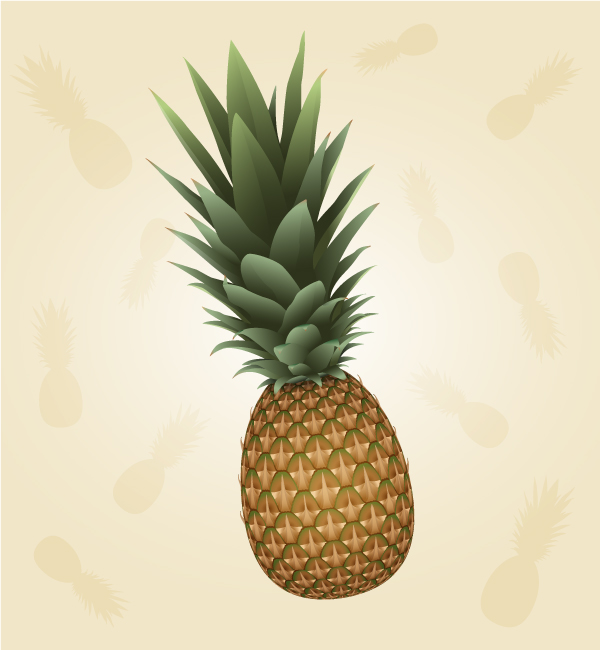# Draw a Realistic Pineapple Using 3D Illustrator Effects

Learn how to easily draw a vector pineapple using the 3D Revolve effect, how to create the realistic texture and apply it with the Map Art option. This tutorial could also by a great way for you to practice your Pen Tool skills by drawing all the different leaves.

## Step 1: Pineapple Base

The first thing to do is to draw the curved path from the image below using the Pen Tool and to give it a 0.5 pt Stroke. The color used is brown (R=157, G=106, B=53).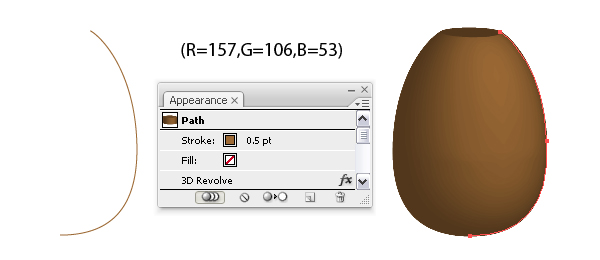## Step 2: 3D Revolve

Now go to Effect > 3D > Revolve and change the values (as shown below) to obtain the pineapple body shape.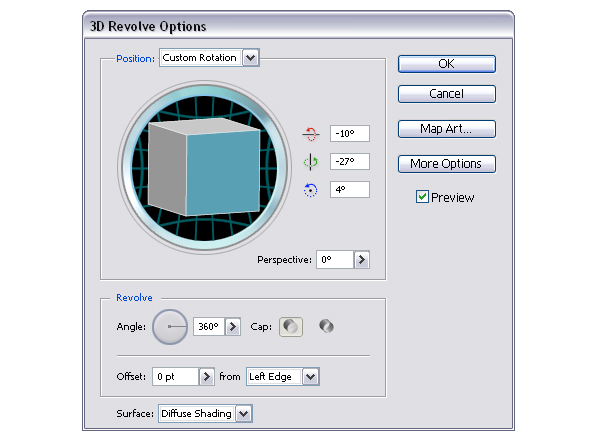## Step 3: Polygons

Here is how to create the texture for the pineapple’s body. Take the Polygon Tool and draw one. Now use the Direct Selection Tool to select just the tow top points and drag them a bit up. Now do the same for the two bottom points. Now go to Effect > Stylize > Round Corners and set a 2 px Radius value.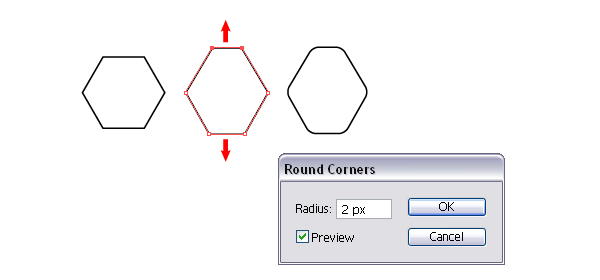## Step 4: Irregular Shape

Go to Object > Path > Add Anchor Points to add the four middle points on each side, then move them by pressing the arrow keys on the keyboard one time in the direction shown in the image for each point. My keyboard increment is set to 1 px.

Fill the shape with a radial gradient using the following colors: dark brown (R=113, G=64, B=19), brown (R=157, G=106, B=53) and green (R=93, G=112, B=43). To give it a more irregular look apply the Scribble Effect that you can find by going to Effects > Stylize.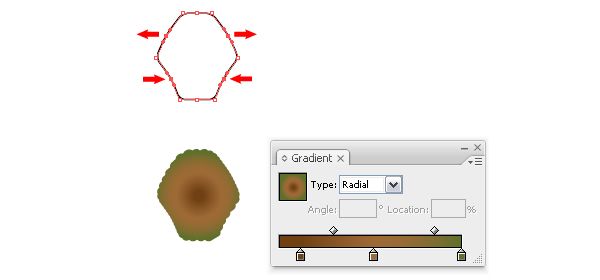## Step 5: Scribble Options

Here is how to change the values in the Scribble Options window. When you are done go to the Object menu and Expand Appearance.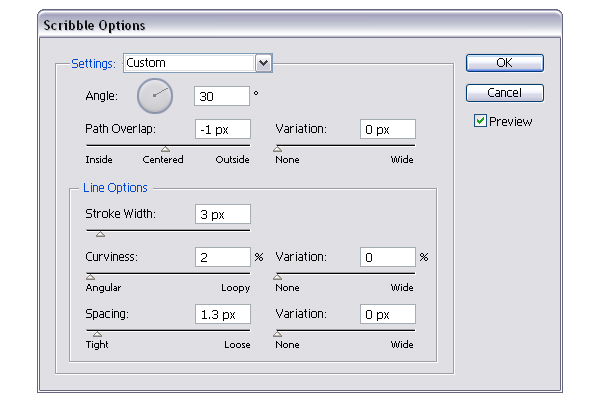## Step 6: Cut and Color

Take the Pen Tool and draw the black shape from below. Only the top portion is important, so draw that first, and then just close the shape at the bottom. Copy and Paste in front the scribble shape made earlier, then select both the black shape and the pasted one, and click Intersect Shape Areas > Expand from the Pathfinder Panel to obtain the second shape on top. Fill it with a radial gradient from one color (R=139, G=86, B=32) to the next (R=237, G=198, B=153).

Copy and Paste in Front this second shape. Using the Direct Selection Tool, move the top point a bit up, then place the shape as shown. Copy and Paste in
Front again the scribble shape and use it to Intersect Shape Areas > Expand as before. Fill the third shape with a radial gradient from one color (R=139, G=86, B=32) to the next (R=211, G=167, B=118).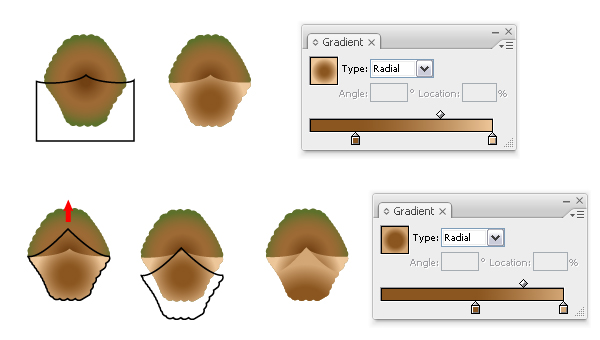## Step 7: Cut and Color More

Now draw the next black shape that you can see below using the Pen Tool, then Copy and Paste in Front again the scribble shape, select them both and Intersect Shape Areas > Expand. Fill the obtained shape with a linear gradient from one color (R=139, G=86, B=32) to the next (R=196, G=157, B=117) and change the angle value to 90 degrees.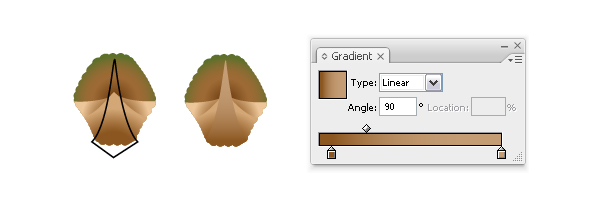## Step 8: Add Another Shape

Do the same to create another shape on top similar to the one made above, but smaller and fill it with a linear gradient from one color (R=157, G=106, B=53) to the next (R=211, G=167, B=118). Change the angle value to 90 degrees this time too.

The last thing to do is to Copy and Paste in Back the first scribble shape made, scale it a bit and change the gradient to a radial one using green (R=93, G=112, B=43) and dark brown (R=97, G=55, B=16).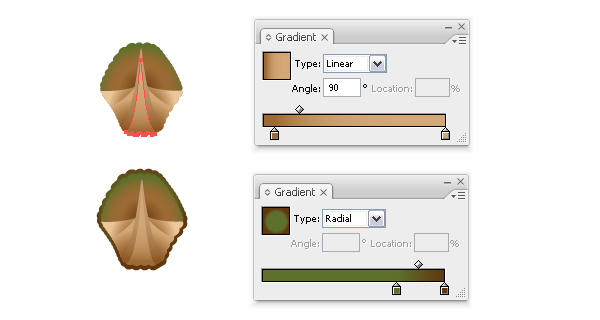## Step 9: Creating the Texture

Now using this group of shapes just made, you should create the entire texture just like the one in step 10, but then the texture will be too complex having so many shapes each one filled with a gradient and Map Art won’t handle it very well. I tried it and I didn’t liked the end result, there was too much quality loss.

So the best way is to simplify the texture and then recolor the shapes later, this way every gradient will look as it should. Fill the shapes with any colors that you want, just a different one for each shape. Then group them all, multiply and arrange them like in the image below with no gaps between them.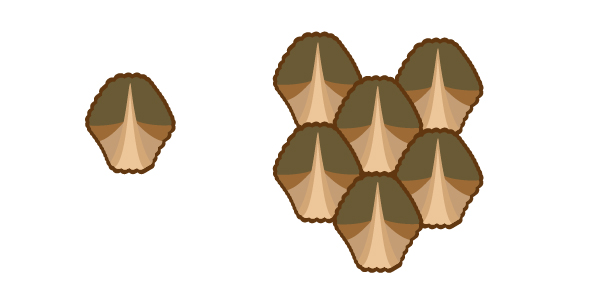## Step 10: Symbols Panel

Here is the entire texture that should be bigger than the pineapple’s body to make sure it will cover it properly. Mine is 17 by 21 rows. When you are done group them all and drag them into the Symbols Panel.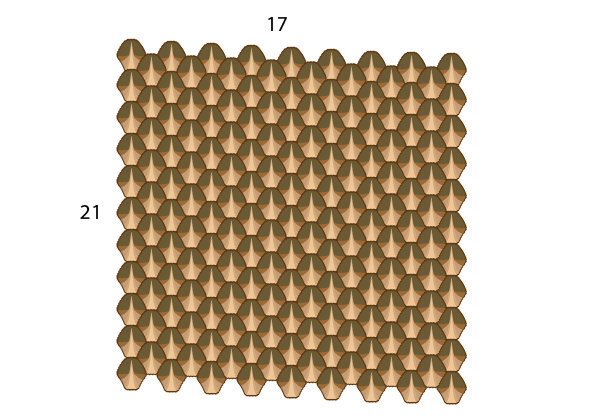## Step 11: Map Art

Select the pineapple body shape, double-click on the 3D Revolve effect applied in the Appearance Panel to open the 3D Revolve Options window, then click on the Map Art button. Select the front surface of the pineapple (mine was 1 of 2) and the symbol texture defined then move it, if needed, until it fits properly on top of the pineapple.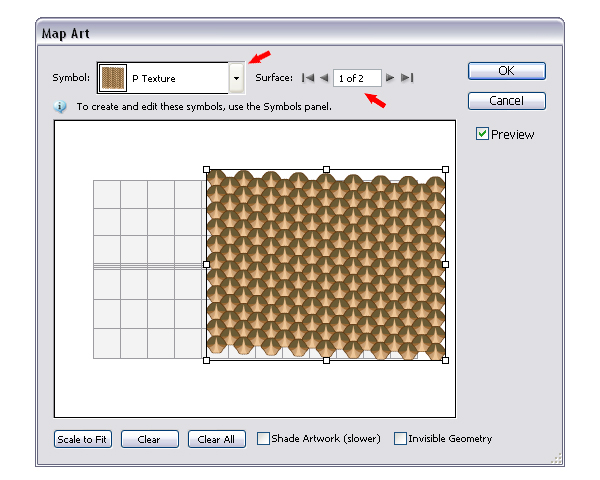## Step 12: Expand and Recolor the Texture

You can see in the next image how the pineapple should look at this point. Go to Object and Expand Appearance and then it’s time to recolor each shape back the way it was first made. This process will take some time but if you select all similar shapes and fill them all at once with the proper gradient specified at the beginning, then select other similar shapes and fill them all at once, and so on you should finish faster.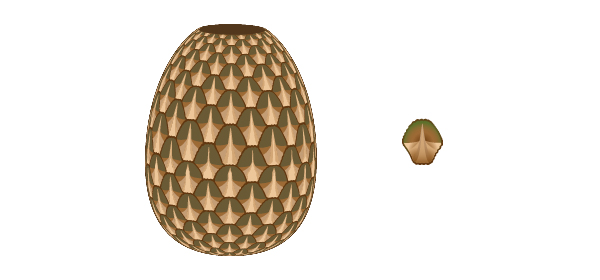## Step 13: Hide the Original Body

Here is how the pineapple should look so far. Also if you zoom and look closely you should see some brown edges all around the pineapple from the original body made at the beginning, so you should delete them or just hide them in the Layers Panel.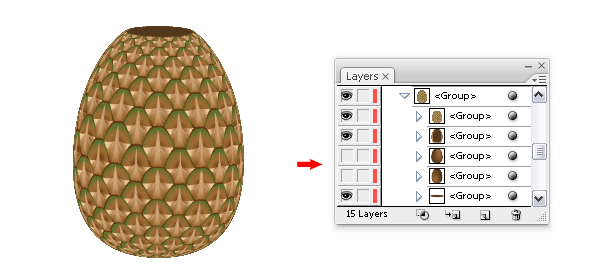## Step 14: Draw and Color Initial Leaves

It’s time to draw the pineapple’s leaves. In the images below you can see some examples of leaves that I picked from all the ones in the final image. Take the Pen Tool and draw a leaf shape like the black one from below, then draw the red one on top.

Only the curved portion is important so you should draw that first and then just simply close the path at the bottom. Now Copy and Paste in Front the black shape, select both the red one and the pasted one and click Intersect Shape Areas > Expand from the Pathfinder Panel to obtain the smaller shape in front.

Fill the first shape drawn with a linear gradient using these colors: brown (R=188, G=125, B=82), light green (R=152, G=177, B=134) and dark green (R=45, G=51, B=27) and also change the angle value, if needed, because the brown color has to be at the tip of the leaf. The smaller shape is filled with a linear gradient from light green (R=152, G=177, B=134) to dark green (R=63, G=71, B=38).

Next draw the other leaf in the image and fill it using the same gradients.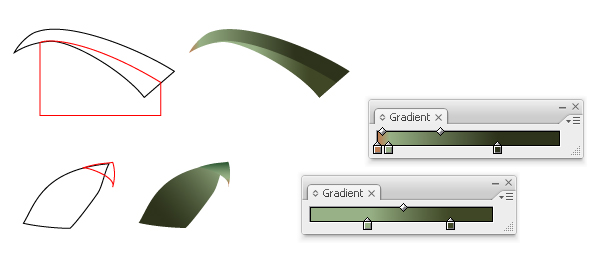## Step 15: Leaves Shaping Up

You can see in the image below some of the first leaves I made similar to the ones above.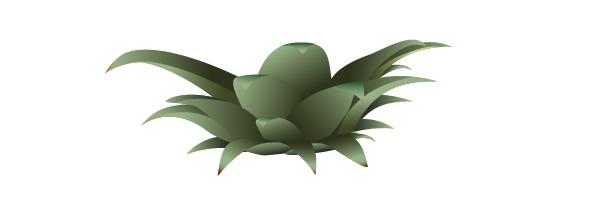## Step 16: Another Leaf Type

Here is another type of leaf for which I used a brighter gradient. The colors are light green (R=190, G=210, B=151) and the same dark green (R=63, G=71, B=38) used before.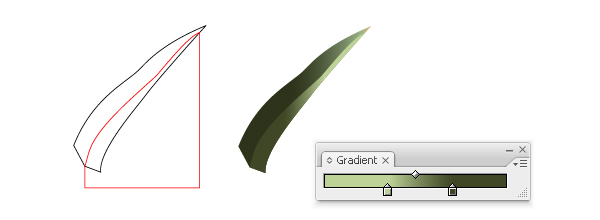## Step 17: Dividing Leaves

Some of the leaves are divided in two pieces and to do that, after you draw the leaf shape take the Add Anchor Point Tool and add a point like in the next image. Then draw the curved path on top starting from the top point to the one added.

It’s important to intersect the top point of the path with the top point from the tip of the leaf, and the last point of the path with the point added on the leaf shape, otherwise the Divide won’t work. Select them both and click Divide in the Pathfinder Panel and you should now have the two pieces of the leaf. Change the gradient angle for one of them to make the difference more visible.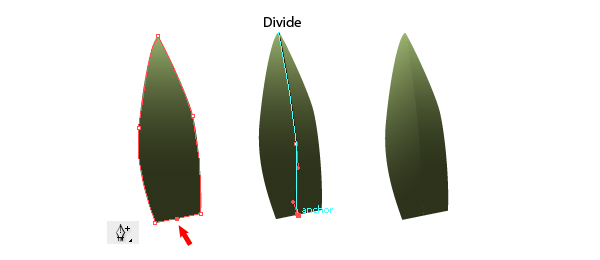## Step 18: Combined Leaves

Continue to draw more leaves until you have something like this. Also you can see them all in the final image.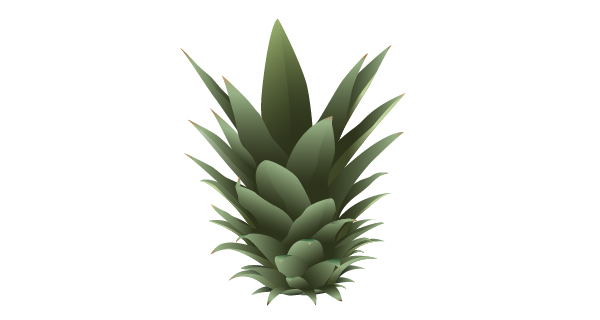There needs to be some shadow under the first leaves drawn, so navigate between all the groups of shapes that are there after expanding the pineapple and you should find a Clipping path. Copy and Paste it in Front, and then draw an oval on top like in the image below. Select both shapes and click Intersect Shape Areas > Expand to obtain the small shape on the right.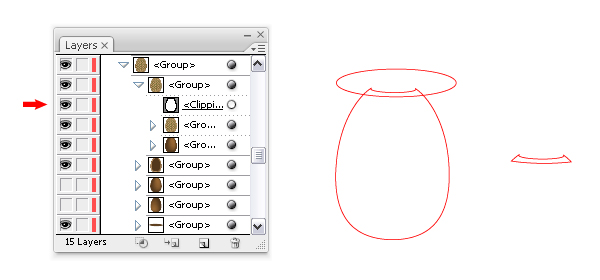## Step 20: Color and Blur the Shadow

Fill the shape with black, go to Effects > Blur > Gaussian Blur and set a 2px Radius value, then lower the Opacity to 50%.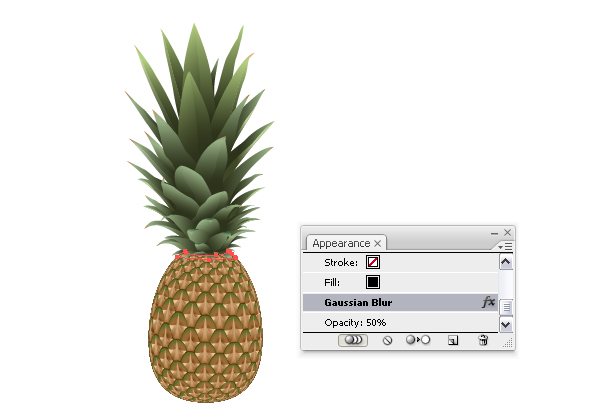## Step 21: Add Small Shapes

The work is almost done. Draw with the Pen Tool a few small shapes like the ones in the next image and place them on the sides of the pineapple’s body. These details add authenticity to the pineapple. The colors used for the gradient are: dark brown (R=54, G=36, B=21), brown (R=139, G=86, B=32) and light brown (R=196, G=157, B=117).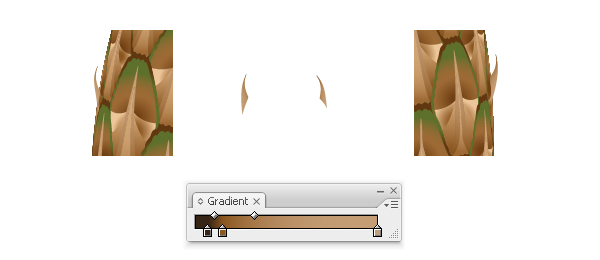The last thing to do is to apply a gradient on top of the pineapple because I think it looks a bit plain without it. Now find again the Clipping path that you used earlier and Paste it in Back (also move it behind the little shadow shape), fill it with a radial gradient that goes from light brown (R=222, G=189, B=152) to dark gray (R=26, G=26, B=26) and change the Blending mode to Soft Light. Adjust the gradient using the Gradient Tool if needed and you are done.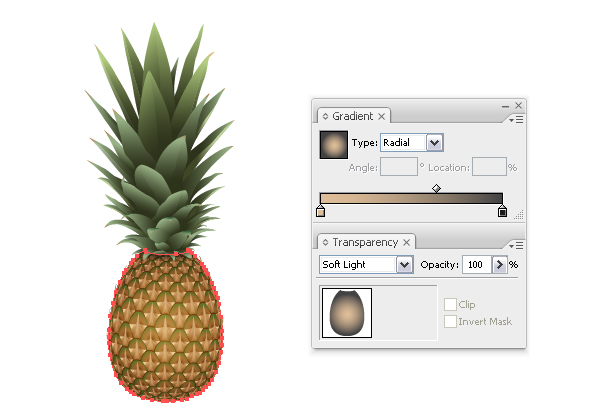## Final Image

Here is the final image of the vector pineapple and I hope you enjoyed this tutorial.